Show Sidebar Hide Sidebar# Matplotlib to plotly in Python

A tutorial on how to convert matplotlib figures to beautiful plotly figures.

## Section 6: Convert your Matplotlib plots to Plotly ¶

Welcome to Plotly's Python API User Guide.

Links to the other sections are on the User Guide's homepage
The Github repository is available here

Section 6 is divided as follows:

Quickstart (make Plotly plot from matplotlib figure object):

>>> import plotly.plotly as py
>>> import matplotlib.pyplot as plt
>>> # auto sign-in with credentials or use py.sign_in()
>>> mpl_fig_obj= plt.figure()
>>> # ... make a matplotlib figure ...
>>> py.plot_mpl(mpl_fig_obj)


The User Guide assumes version 1.0 and up of Plotly's Python API.

# (*) Import plotly package
import plotly

plotly.__version__

'1.6.6'


##### Matplotlib + Plotly = Matplotlylib

The matplotlylib project is a collaboration between Plotly , mpld3 and Jake Vanderplas that lets you convert matplotlib figures to beautiful D3.js graphs with Plotly's Python API.

The goal of mpld3 is to expand Python's matplotlib plotting package use to the browser. Now, together with Plotly, users can share their plots and data sets online, for free.

While Plotly's Python API gives users access to Plotly directly, users may already have high-quality plots that they want to get online and share. Standing on the shoulders of the mplexporter , matplotlylib allows users to convert matplotlib figures to Plotly figures with a simple function call.

If your data sets are important to you, they are important to us, and we will help you share them with others. As a bonus, you will be able to use Plotly's awesome interactive web GUI.

This section showcases the current state of matplotlylib and how you can incorporate it into your workflow.

We first import a few modules and sign in to Plotly using our credentials file

# (*) To communicate with Plotly's server, sign in with credentials file
import plotly.plotly as py

# (*) Useful Python/Plotly tools
import plotly.tools as tls

# (*) Graph objects to piece together plots
from plotly.graph_objs import *

import numpy as np  # (*) numpy for math functions and arrays


If you are not familiar with credentials files, refer to the User Guide's homepage .

We also need to import matplotlib and let's turn on the IPython inline magic to display matplotlib plots directly inside this notebook.

import matplotlib.pyplot as plt # (*) import matplotlib

%matplotlib inline


### 6.1 Matplotlib to Plotly conversion basics ¶

To convert a matplotlib figure to a Plotly figure, Python API users now have, in version 1.0, the following three functions made available to them:

•  tls.mpl_to_plotly  : takes in a matplotlib figure object (i.e.  mpl_fig = plt.figure()  ) and returns a Plotly figure object

•  py.plot_mpl  : takes in a matplotlib figure object and creates a Plotly plot at a unique URL, and optionally opens a new browser tab at this URL.

•  py.iplot_mpl  : similar to  py.plot_mpl  but for IPython notebook use. It displays the returned Plotly figure directly in the IPython notebook in session.

So, let's get started by generating this damped oscillation graph using matplotlib. The following code cell has been adapted from the matplotlib example bank. Thanks!

# Package all mpl plotting commands inside one function
def plot_mpl_fig():

# Make two time arrays
t1 = np.arange(0.0, 2.0, 0.1)
t2 = np.arange(0.0, 2.0, 0.01)

# N.B. .plot() returns a list of lines.
# The "l1, = plot" usage extracts the first element of the list
# into l1 using tuple unpacking.
# So, l1 is a Line2D instance, not a sequence of lines
l1, = plt.plot(t2, np.exp(-t2), label='decaying exp.')
l2, l3 = plt.plot(t2, np.sin(2 * np.pi * t2), '--go',
t1, np.log(1 + t1), '.')
l4, = plt.plot(t2, np.exp(-t2) * np.sin(2 * np.pi * t2), 'rs-.')

# Add axis labels and title
plt.xlabel('time')
plt.ylabel('volts')
plt.title('Damped oscillation')

return (l1, l2, l3, l4)  # return line objects (for legend, later)

# Plot it!
plot_mpl_fig()

# N.B. get matplotlib figure object and assign a variable to it
mpl_fig1 = plt.gcf()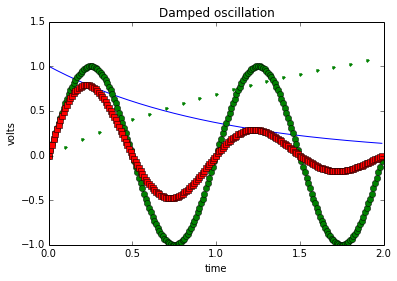Note that in the above we retrieved the matplotlib figure object after generating the plot, using

plt.gcf()


where  .gcf()  stands for get current figure. Equivalently, we can use

plt.figure()


to initialize the figure object before the plotting commands. The latter method is used in this section from this point on.

##### Convert a matplotlib figure object to a Plotly figure object ¶

Let's first try out the  tls.mpl_to_plotly  function.

As always, a good way to start is to run help on the object in question:

help(tls.mpl_to_plotly)

Help on function mpl_to_plotly in module plotly.tools:

mpl_to_plotly(fig, resize=False, strip_style=False, verbose=False)
Convert a matplotlib figure to plotly dictionary and send.

All available information about matplotlib visualizations are stored
within a matplotlib.figure.Figure object. You can create a plot in python
using matplotlib, store the figure object, and then pass this object to
the fig_to_plotly function. In the background, mplexporter is used to
crawl through the mpl figure object for appropriate information. This
information is then systematically sent to the PlotlyRenderer which
creates the JSON structure used to make plotly visualizations. Finally,
these dictionaries are sent to plotly and your browser should open up a
new tab for viewing! Optionally, if you're working in IPython, you can
set notebook=True and the PlotlyRenderer will call plotly.iplot instead
of plotly.plot to have the graph appear directly in the IPython notebook.

Note, this function gives the user access to a simple, one-line way to
render an mpl figure in plotly. If you need to trouble shoot, you can do
this step manually by NOT running this fuction and entereing the following:

===========================================================================
from mplexporter import Exporter
from mplexporter.renderers import PlotlyRenderer

# create an mpl figure and store it under a varialble 'fig'

renderer = PlotlyRenderer()
exporter = Exporter(renderer)
exporter.run(fig)
===========================================================================

You can then inspect the JSON structures by accessing these:

renderer.layout -- a plotly layout dictionary
renderer.data -- a list of plotly data dictionaries

Positional arguments:
fig -- a matplotlib figure object
api_key -- a valid api_key for the above username **
notebook -- an option for use with an IPython notebook

** Don't have a username/api_key? Try looking here:
https://plot.ly/plot

** Forgot your api_key? Try signing in and looking here:
https://plot.ly/python/getting-started



So, we convert the matplotlib figure object of our previous figure to a Plotly figure object, with verbose turned on just for fun:

py_fig1 = tls.mpl_to_plotly(mpl_fig1, verbose=True)

Initialized PlotlyRenderer
Opening figure
Opening axes
Attempting to draw a line ... with just lines
Heck yeah, I drew that line
Attempting to draw a line ... with both lines+markers
Heck yeah, I drew that line
Attempting to draw a line ... with just markers
Heck yeah, I drew that line
Attempting to draw a line ... with both lines+markers
Heck yeah, I drew that line
Attempting to draw an mpl text object
Text object is an xlabel
Attempting to draw an mpl text object
Text object is a ylabel
Attempting to draw an mpl text object
Text object is a title
Attempting to draw a title
Only one subplot found, adding as a plotly title
Closing axes
Closing figure



Now, print the yielded Plotly figure object:

print(py_fig1.to_string())

Figure(
data=Data([
Scatter(
x=[0.0, 0.01, 0.02, 0.029999999999999999, 0.040000000000000001,  ],
y=[1.0, 0.99004983374916811, 0.98019867330675525, 0.970445533.., ],
mode='lines',
name='decaying exp.',
line=Line(
color='#0000FF',
width=1.0,
dash='solid',
opacity=1
),
xaxis='x1',
yaxis='y1'
),
Scatter(
x=[0.0, 0.01, 0.02, 0.029999999999999999, 0.040000000000000001,  ],
y=[0.0, 0.062790519529313374, 0.12533323356430426, 0.18738131.., ],
mode='lines+markers',
name='_line1',
marker=Marker(
color='#007F00',
size=6,
symbol='dot',
line=Line(
color='#000000',
width=0.5
),
opacity=1
),
line=Line(
color='#007F00',
width=1.0,
dash='dash',
opacity=1
),
xaxis='x1',
yaxis='y1'
),
Scatter(
x=[0.0, 0.10000000000000001, 0.20000000000000001, 0.300000000.., ],
y=[0.0, 0.095310179804324935, 0.18232155679395459, 0.26236426.., ],
mode='markers',
name='_line2',
marker=Marker(
color='#007F00',
size=6,
symbol='dot',
line=Line(
color='#007F00',
width=0.5
),
opacity=1
),
xaxis='x1',
yaxis='y1'
),
Scatter(
x=[0.0, 0.01, 0.02, 0.029999999999999999, 0.040000000000000001,  ],
y=[0.0, 0.062165743421020596, 0.12285146926097672, 0.18184335.., ],
mode='lines+markers',
name='_line3',
marker=Marker(
color='#FF0000',
size=6,
symbol='square',
line=Line(
color='#000000',
width=0.5
),
opacity=1
),
line=Line(
color='#FF0000',
width=1.0,
dash='dashdot',
opacity=1
),
xaxis='x1',
yaxis='y1'
)
]),
layout=Layout(
title='Damped oscillation',
titlefont=Font(
size=12.0,
color='#000000'
),
showlegend=False,
autosize=False,
width=480,
height=320,
margin=Margin(
l=60,
r=47,
b=40,
t=31,
),
hovermode='closest',
xaxis1=XAxis(
title='time',
titlefont=Font(
size=10.0,
color='#000000'
),
range=[0.0, 2.0],
domain=[0.0, 1.0],
type='linear',
showgrid=False,
zeroline=False,
showline=True,
nticks=5,
ticks='inside',
tickfont=Font(
size=10.0
),
mirror='ticks',
anchor='y1',
side='bottom'
),
yaxis1=YAxis(
title='volts',
titlefont=Font(
size=10.0,
color='#000000'
),
range=[-1.0, 1.5],
domain=[0.0, 1.0],
type='linear',
showgrid=False,
zeroline=False,
showline=True,
nticks=6,
ticks='inside',
tickfont=Font(
size=10.0
),
mirror='ticks',
anchor='x1',
side='left'
)
)
)



And there it is. A full Plotly figure object corresponding to our matplotlib plot!

Even though the matplotlib plot only has one x-axis and one y-axis, matplotlylib converted the matplotlib axes to  'xaxis1'  and  'yaxis1'  keys in the Plotly layout object, consistent with figure object containing subplots (more in subsection 6.3 ).

Moreover, note that  tls.mpl_to_plotly  comes with a few optional keyword arguments. Namely,  resize  and  strip_style  which give users some flexibiliy in the conversion.

By default, the converted Plotly figure object keeps the original matplotlib figure's size in pixels. These specifications are linked to the  'layout'  key of the Plotly figure object. More specifically for our first figure:

# Print key-value pairs corresponding to the figure's size
for i in ['autosize', 'width', 'height']:
print i, py_fig1['layout'][i]

autosize False
width 480
height 320



Then, setting  resize  to  True  in the  tls.mpl_to_plotly  call effectively deletes the three above keys (as the  .pop()  dictionary method would do). In this case, the converted Plotly figure object acquires Plotly's auto-size functionality when plotted.

The  strip_style  argument allows users to convert a matplotlib figure to Plotly's beautiful default style settting without having to manipulation the Plotly figure object. The  strip_style  argument is in display next.

##### Plot a matplotlib figure in Plotly ¶

Start by running help on  py.iplot_mpl  (or  py.plot_mpl  ),

help(py.iplot_mpl)

Help on function iplot_mpl in module plotly.plotly.plotly:

iplot_mpl(fig, resize=True, strip_style=False, update=None, **plot_options)
Replot a matplotlib figure with plotly in IPython.

This function:
1. converts the mpl figure into JSON (run help(plolty.tools.mpl_to_plotly))
2. makes a request to Plotly to save this figure in your account
3. displays the image in your IPython output cell

Positional agruments:
fig -- a figure object from matplotlib

Keyword arguments:
resize (default=True) -- allow plotly to choose the figure size
strip_style (default=False) -- allow plotly to choose style options
update (default=None) -- update the resulting figure with an 'update'
dictionary-like object resembling a plotly 'Figure' object

plot_options -- run help(plotly.plotly.iplot)



Note that  resize  keyword argument is set to  True  by default in  py.iplot_mpl  (N.B. and for  py.plot_mpl  ). unlike in  tls.mpl_to_plotly  .

Next, we send our matplotlib figure object to  py.iplot_mpl  , give it a file name as keyword argument likewise to  py.iplot  and  py.plot  . This results in:

py.iplot_mpl(mpl_fig1, filename='s6_damped_oscillation')


Wow! The difference between a matplotlib plot and a Plotly plot is merely one line of code.

Our plot has now a unique URL and can, along with the underlying data sets, be shared online. As with all Plotly plots, you can hover, zoom, and pan on the figure. Fantastic.

Plotly's Python API makes it easy to strip a figure's style options which, in essence, resets all style keys to the beautiful-looking Plotly defaults. This can be done in several ways adapted to your personal workflow. First, by adding a keyword argument in the  py.iplot_mpl  or  py_plot_mpl  call:

# (1) Plot mpl figure object as a Plotly plot with default style options
py.iplot_mpl(mpl_fig1, strip_style=True,
filename='s6_damped_oscillation-default-style')


Nice!

Notice that Plotly caught the  label  keyword argument in the first  plt.line  call in our  plot_mpl_fig  funciton. This label is translated to the  'name'  key in the corresponding trace object; hence, it is displayed on hover and in the legend (as  showlegend  is set to  True  by default in Plotly). The other lines which were defined without a  label  keyword argument are named sequencially with a leading underscore in the same order as in the original matplotlib plot.

If more convenient for you, the two following code cells yield the same Plotly plot as the above. You are invited to copy and paste the URLs in your browser to check the results.

# (2) Strip style in convertion for mpl fig obj to plotly fig obj, plot
py_fig1_ss = tls.mpl_to_plotly(mpl_fig1, strip_style=True)

py.plot(py_fig1_ss, filename='s6_damped_oscillation-default-style2',
auto_open=False)

u'https://plot.ly/~etpinard/229'

# (3) Strip style in retruned plotly fig obj, plot
py_fig1.strip_style()

py.plot(py_fig1, filename='s6_damped_oscillation-default-style3',
auto_open=False)

u'https://plot.ly/~etpinard/230'

##### Careful, matplotlib is not perfect (yet) ¶

Please note that, in the current version of matplotlylib, matplotlib legends do not fully convert to Plotly legends.

For example,

# Set new mpl figure object
mpl_fig2 = plt.figure()

# Replot first mpl figure, add legend
(l1, l2, l3, l4) = plot_mpl_fig()
plt.legend((l2, l4), ('oscillatory', 'damped'), 'upper right')

<matplotlib.legend.Legend at 0x7f18d267a8d0>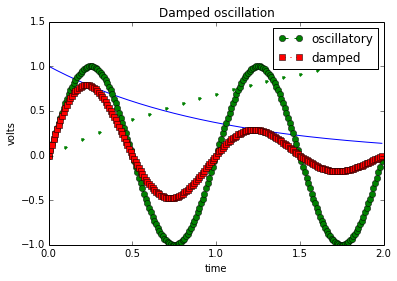# Plot mpl figure object as a Plotly plot
py.iplot_mpl(mpl_fig2, filename='s6_damped_oscillation-legend')

/usr/local/lib/python2.7/dist-packages/plotly/matplotlylib/renderer.py:382: UserWarning:

Bummer! Plotly can currently only draw Line2D objects from matplotlib that are in 'data' coordinates!

/usr/local/lib/python2.7/dist-packages/plotly/matplotlylib/renderer.py:479: UserWarning:

I found a path object that I don't think is part of a bar chart. Ignoring.



Legends are treated as simple text annotations.

That said, once you have the Plotly figure in hand, it is pretty straight forward to add a Plotly legend to your Plotly plot:

# Convert mpl fig obj to plotly fig obj, resize to plotly's default
py_fig2 = tls.mpl_to_plotly(mpl_fig2, resize=True)

# Delete misplaced legend annotations
py_fig2['layout'].pop('annotations', None)

# Add legend, place it at the top right corner of the plot
py_fig2['layout'].update(
showlegend=True,
legend=Legend(
x=1.05,
y=1
)
)

# Send updated figure object to Plotly, show result in notebook
py.iplot(py_fig2, filename='s6_damped_oscillation-legend2')


Again, Plotly caught the  label  keyword from the  plt.line  call.

To make it look like the original matplotlib plot, we need to add a few key-value pairs to the trace objects:

# Add name to be shown on hover and legend for two of the traces
py_fig2['data'].update(name='oscillatory')
py_fig2['data'].update(name='damped')

# Do not include the remaining traces in legend
py_fig2['data'].update(showlegend=False)
py_fig2['data'].update(showlegend=False)

# Send updated figure object to Plotly, show result in notebook
py.iplot(py_fig2, filename='s6_damped_oscillation-legend3')


This is much better than the orginal matplotlib figure, right?

The previous updates can be made by passing a single keyword argument to  py.iplot_mpl  :

# Update dictionary
update = dict(
layout=dict(
annotations=[dict(text=' ')],  # blank text in legend 'annotation'
showlegend=True,               # show legend
legend=Legend(
x=1.05,     # legend position
y=1
)
),
data=[
dict(showlegend=False),   # do not show trace 1 in legend
dict(name='oscillatory'), # legend label for trace 2
dict(showlegend=False),   # do not show trace 3 in legend
dict(name='damped')       # legend label for trace 4
]
)

# Plot mpl figure object as a Plotly plot, with update dictionary
py.iplot_mpl(mpl_fig2, resize=True, update=update,
filename='s6_damped_oscillation-legend4')


Thank you Adam Hughes for the suggestion.

### 6.2 Supported plot types ¶

So far, matplotlylib supports convertions of:

Here are a couple examples:

##### Bar Charts ¶
mpl_fig_bar = plt.figure()  # set new mpl figure object

# Locations and height of the bars
locs = [1, 2, 3, 4, 5, 6, 7]
height = [5, 6, 1, 4, 3, 10, 15]

# Plot mpl bar chart, bars have a width of 1 unit
ax.bar(locs, height, width=1)

<Container object of 7 artists>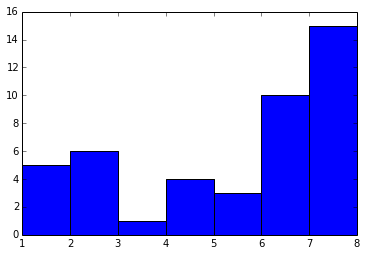# Convert mpl figure object to Plotly plot
py.iplot_mpl(mpl_fig_bar, filename='s6_bars')


Recall that, by default,  py.plot_mpl  and  py.iplot_mpl  resize the inputted matplotlib figure object.

##### Histograms ¶
mpl_fig_hist = plt.figure()  # set new mpl figure objects

N = 10000  # sample size

y = np.random.randn(N)  # normally distribution random numbers

ax.set_xlabel(r"random variable $X$")  # set axis labels
ax.set_ylabel(r"$\mathcal{P}(X)$")     #  using LaTeX symbols

ax.annotate('Sample size: ' + str(N),
textcoords='axes fraction',
xy=(0.7,0.9), xytext=(0.7,0.9))

# Plot mpl histogram with 100 bins
out = ax.hist(y, bins=100)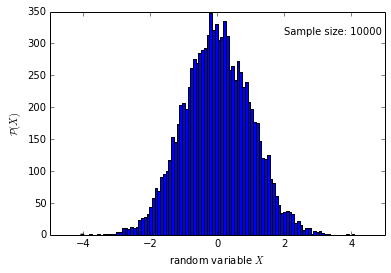# Convert mpl figure object to Plotly plot
py.iplot_mpl(mpl_fig_hist, filename='s6_histograms')


Nice!

Matplotlylib is able to convert $\LaTeX$ symbols from matplotlib figure objects as well as text annotations with ease.

Please note that matplotlylib converts matplotlib histograms into Plotly  Bar  objects (not into Plotly  Histogram  objects). More clearly,

# Convert mpl figure object to plotly
py_fig_hist = tls.mpl_to_plotly(mpl_fig_hist)

# Print the yielded plotly figure object
print(py_fig_hist.to_string())

Figure(
data=Data([
Bar(
x=[-4.0300728711721483, -3.9484305111402387, -3.8667881511083.., ],
y=[1.0, 0.0, 0.0, 1.0, 0.0, 0.0, 2.0, 0.0, 2.0, 1.0, 1.0, 2.0,   ],
orientation='v',
marker=Marker(
color='#0000FF',
line=Line(
width=1.0
)
),
opacity=1,
xaxis='x1',
yaxis='y1'
)
]),
layout=Layout(
showlegend=False,
autosize=False,
width=480,
height=320,
annotations=Annotations([
Annotation(
x=0.6981233243967828,
y=0.8963855421686748,
xref='paper',
yref='paper',
text='Sample size: 10000',
showarrow=False,
font=Font(
size=10.0,
color='#000000'
),
xanchor='left',
yanchor='bottom',
align='left',
opacity=1
)
]),
margin=Margin(
l=60,
r=47,
b=40,
t=31,
),
hovermode='closest',
bargap=0.0,
xaxis1=XAxis(
title='random variable $X$',
titlefont=Font(
size=10.0,
color='#000000'
),
range=[-5.0, 5.0],
domain=[0.0, 1.0],
type='linear',
showgrid=False,
zeroline=False,
showline=True,
nticks=7,
ticks='inside',
tickfont=Font(
size=10.0
),
mirror='ticks',
anchor='y1',
side='bottom'
),
yaxis1=YAxis(
title='$\\mathcal{P}(X)$',
titlefont=Font(
size=10.0,
color='#000000'
),
range=[0.0, 350.0],
domain=[0.0, 1.0],
type='linear',
showgrid=False,
zeroline=False,
showline=True,
nticks=8,
ticks='inside',
tickfont=Font(
size=10.0
),
mirror='ticks',
anchor='x1',
side='left'
)
)
)



That is, the computation required to plot a histogram from a set of values is not made twice.

##### Log scales ¶

The following plot was inspired by the log demo of the matplotlib example bank.

mpl_fig_log = plt.figure()  # set new mpl figure object

# A simple exponential y=x^2
x = 10.0**np.linspace(0.0, 6.0, 20)
y = x**2.0

plt.loglog(x,y) # plot as log-log

# Add title, axis labels and grid
plt.title('A log-log plot')
ax.set_xlabel('log x-axis')
ax.set_ylabel('log y-axis')
plt.grid(True)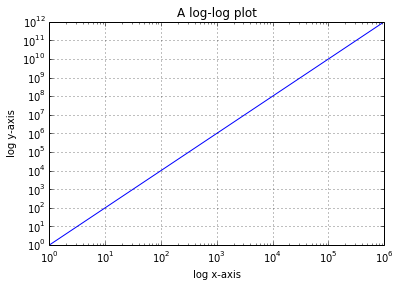# Convert mpl figure object to Plotly plot
py.iplot_mpl(mpl_fig_log, filename='s6_log-scales')


The tick labels on the Plotly figure are not exactly the same as on the matplotlib figure. Hopefully, you agree that the converted tick labels look better than the original.

##### Bubble Charts ¶

The following example was inspired by this post on the Glowing Python blog. Thanks!

import urllib2       # (*) urllib2 for in-session downloads
import pandas as pd  # (*) pandas for dataframe manipulation

# Import data file from URL into pd datafram in session
data_url = 'http://datasets.flowingdata.com/crimeRatesByState2005.csv'
data_file = urllib2.urlopen(data_url)

df = df.drop(df.index)  # drop first row (US totals)
df = df[df['murder'] < 11] # drop out-of-range rows

mpl_fig_bubble = plt.figure()         # (!) set new mpl figure object

plt.axis([0,11,200,1280])
plt.xlabel('Murders per 100,000 population')
plt.ylabel('Burglaries per 100,000 population')

scatter = ax.scatter(
df['murder'],
df['burglary'],
c=df['larceny_theft'],        # using some color scale
s=np.sqrt(df['population']),
linewidths=2,
edgecolor='w',
alpha=0.6
)

for i_X, X in df.iterrows():
plt.text(
X['murder'],
X['burglary'],
X['state'][0:8], # only the first 8 letters
size=8,
horizontalalignment='center'
)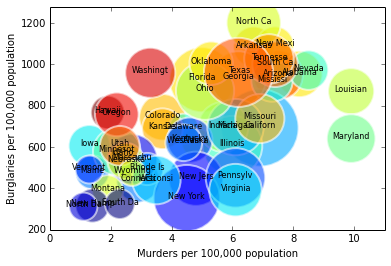# Convert mpl figure object to Plotly plot
py.iplot_mpl(mpl_fig_bubble, filename='s6_bubble-chart')


For another matplotlylib examples, refer to our online documentation .

### 6.3 Converting subplots ¶

Matplotlylib also supports subplot conversions.

Again, here are a couple examples:

mpl_fig_splt1 = plt.figure()  # set a new mpl figure object

t = np.arange(0.0, 1.0, 0.01)  # some time array

# Make top subplot
ax1.plot(t, np.sin(2*np.pi*t), label='1 Hz')    # add data, with label
ax1.grid(True)                                  # put grid
ax1.set_ylim((-2,2))                            # y-axis limit

# Similarly for bottom subplot
ax2.plot(t, np.sin(2*2*np.pi*t), label='2 Hz')
ax2.grid(True)
ax2.set_ylim((-2,2))

# Set plot title and x-axis title
ax1.set_title('Two sine waves')
l = ax2.set_xlabel('time [s]')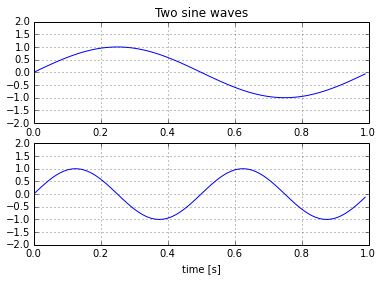py.iplot_mpl(mpl_fig_splt1, filename='s6_subplots1')


By default, matplotlib subplots don't have common axis elements and so the rendered Plotly plot will reflect this (try panning one of the axes!).

However, by first converting the matplotlib figure object to a Plotly figure object, we can easily make a shared-axis version of the above plot.

# Convert mpl figure object to Plotly figure object with default style and size
py_fig_splt1 = tls.mpl_to_plotly(mpl_fig_splt1, strip_style=True, resize=True)

# Print the axes' domains
for i in ['xaxis1', 'yaxis1', 'xaxis2', 'yaxis2']:
print(i, py_fig_splt1['layout'][i]['domain'])

xaxis1 [0.0, 1.0]
yaxis1 [0.54545454545454553, 1.0]
xaxis2 [0.0, 1.0]
yaxis2 [0.0, 0.45454545454545453]



Notice the  'yaxis1'  (N.B. not  'yaxis'  ) is above  'yaxis2'  which is consistent with our previous matplotlib commands. That said, it is not consistent with  tls.get_subplots  .

Next, we delete  'xaxis2'  and reassign the each trace to the correct axes.

# Delete 'xaxis2' key
py_fig_splt1['layout'].pop('xaxis2', None)

# Delete 'anchor' keys, they have no effet with 'xaxis2'
for i in ['yaxis1', 'yaxis2']:
py_fig_splt1['layout'][i].pop('anchor', None)

# Link both traces to 'xaxis1'
py_fig_splt1['data'].update(xaxis='x1')
py_fig_splt1['data'].update(xaxis='x1')

# Link top trace to 'yaxis1' (as in original mpl figure)
#  and bottom trace to 'yaxis2'
py_fig_splt1['data'].update(yaxis='y1')
py_fig_splt1['data'].update(yaxis='y2')

# Send updated figure object to Plotly, show result in notebook
py.iplot(py_fig_splt1, filename='s6_subplots1-shared-xaxis')


Matplotlylib can convert more evolved subplot grids as well:

# For a more precise subplot layout
import matplotlib.gridspec as gridspec

mpl_fig_splt2 = plt.figure()  # set new mpl figure object

# On a 3x3 subplot grid
gs = gridspec.GridSpec(3, 3)
gs.update(wspace=0.5, hspace=0.5)  # vertical and horizontal spacing

# Subplot 1, top over all columns
ax1.plot([1, 2, 3, 4, 5], [10, 5, 10, 5, 10], 'r-', label='sbplt 1')

# Subplot 2, middle height over 2 left-most columns
ax2.plot([1, 2, 3, 4], [1, 4, 9, 16], 'k-', label='sbplt 2')

# Subplot 3, left over 2 bottom-most rows
ax3.plot([1, 2, 3, 4], [1, 10, 100, 1000], 'b-', label='sbplt 3')

# Subplot 4, bottom-left
ax4.plot([1, 2, 3, 4], [0, 0, 1, 1], 'g-', label='sbplt 4')

# Subplot 5, bottom-center
ax5.plot([1, 2, 3, 4], [1, 0, 0, 1], 'c-', label='sbplt 5')

[<matplotlib.lines.Line2D at 0x7f18d0153a10>]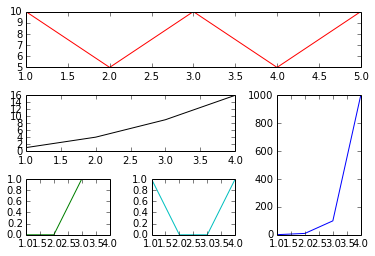# Plot as Plotly plot
py.iplot_mpl(mpl_fig_splt2, filename='s6_subplots2')

# Plot as Plotly plot with default style
py.iplot_mpl(mpl_fig_splt2, strip_style=True, filename='s6_subplots2-default')


Nice!

Again, if you're fond of matplotlib's syntax, or, you are just feeling like dipping your toes in the Plotly pool for now, a simple call to  py.plot_mpl  or  py.iplot_mpl  is all you need.

### 6.4 The nitty gritty of matplotlylib ¶

Out of curiosity or for the sake of troubleshooting, you might find yourself needing to inspect the dictionaries and possibly even the mplexporter. The following shows how you go about running the PlotlyRenderer and making a manual call to Plotly.

mpl_fig_ng = plt.figure()         # set new mpl figure obj.

ax.plot([10, 20, 30], [100, 200, 300], 'bo')  # add a few pts too plot

ax.set_xlim(5, 35)    # set axes limits
ax.set_ylim(90, 310)

(90, 310)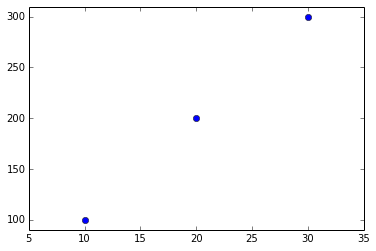from plotly.matplotlylib import Exporter, PlotlyRenderer

renderer = PlotlyRenderer()    # make shorcuts
exporter = Exporter(renderer)


The Exporter crawls through the matplotlib figure, judiciously grabs pertinent information, and invokes the renderer with which it was instantiated. The PlotlyRenderer accepts calls from the Exporter and creates an appropriate JSON dictionary for use with Plotly. So,

# Grab info from matplotlib figure
exporter.run(mpl_fig_ng)

# Create JSON dictionary for use with Plotly
renderer.plotly_fig

{'data': [{'marker': {'color': '#0000FF',
'line': {'color': '#000000', 'width': 0.5},
'opacity': 1,
'size': 6,
'symbol': 'dot'},
'mode': 'markers',
'name': '_line0',
'type': u'scatter',
'x': [10.0, 20.0, 30.0],
'xaxis': 'x1',
'y': [100.0, 200.0, 300.0],
'yaxis': 'y1'}],
'layout': {'autosize': False,
'height': 320,
'hovermode': 'closest',
'margin': {'b': 40, 'l': 60, 'pad': 0, 'r': 47, 't': 31},
'showlegend': False,
'width': 480,
'xaxis1': {'anchor': 'y1',
'domain': [0.0, 1.0],
'mirror': 'ticks',
'nticks': 7,
'range': [5.0, 35.0],
'showgrid': False,
'showline': True,
'side': 'bottom',
'tickfont': {'size': 10.0},
'ticks': 'inside',
'type': 'linear',
'zeroline': False},
'yaxis1': {'anchor': 'x1',
'domain': [0.0, 1.0],
'mirror': 'ticks',
'nticks': 7,
'range': [90.0, 310.0],
'showgrid': False,
'showline': True,
'side': 'left',
'tickfont': {'size': 10.0},
'ticks': 'inside',
'type': 'linear',
'zeroline': False}}}


OK.

Finally, to create the figure in Plotly, call  py.iplot  :

py.iplot(renderer.plotly_fig, filename='s6_nitty-gritty')


Additionally, you can use this opportunity to add to or modify the data and layout dictionaries. Just as an example, we can change the plot and paper background colors:

# Use Plotly's default auto-size
renderer.resize()

# Add a few layout features to the figure object
renderer.plotly_fig['layout']['paper_bgcolor'] = '#fefafd'

# Send updated figur object
py.iplot(renderer.plotly_fig, filename='s6_nitty-gritty2')


#### Go to User Guide's homepage